# Growth predictions in biogrowth

library(biogrowth)
library(tidyverse)
#> ── Attaching packages ─────────────────────────────────────── tidyverse 1.3.0 ──
#> ✓ ggplot2 3.3.5     ✓ purrr   0.3.4
#> ✓ tibble  3.1.6     ✓ dplyr   1.0.8
#> ✓ tidyr   1.2.0     ✓ stringr 1.4.0
#> ✓ readr   2.1.2     ✓ forcats 0.5.0
#> ── Conflicts ────────────────────────────────────────── tidyverse_conflicts() ──
#> x dplyr::lag()    masks stats::lag()

## The function predict_growth()

Growth predictions in biogrowth are done using the predict_growth() function. It provides a unified interface for making predictions with a variety of modeling approaches. This vignette provides a detailed description of the arguments of predict_growth() and how they can be used to make different kinds of model predictions. For a detailed description of the mathematical models available in the package and the computational methods used, please check the relevant vignette.

The predict_growth() function has 6 arguments, as well as the ... argument to pass additional arguments to the functions used for making the predictions. The prediction is defined by the following 6 arguments:

• times defines the time points for the calculation of the prediction,
• primary_model defines the primary growth model (both the model equation an the values of the model parameters),
• environment defines the type of environment. If environment="constant", the predictions are calculated using only a primary model. If environment="dynamic", the function accounts for the effect of variations in the environmental conditions in the specific growth rate through secondary models.
• secondary_models defines the secondary growth models for each environmental factor.
• env_conditions defines the variation of the environmental conditions during the experiment.
• formula defines the column name in env_conditions used to state the elapsed time. By default, formula = . ~ time indicating that the column is named time.

In the following sections, we will describe how these arguments can define different modeling approaches.

Apart from these arguments, the function includes check. When check=TRUE (default), the function performs several checks of the models (names of the parameters, completeness of the model…) before doing any calculation, returning a warning or error if any inconsistency is detected.

Because the growth of population often spans several orders of magnitude, the models implemented in biogrowth are often described in terms of logarithms. The predict_growth() function allows the definition of different bases for the logarithm both for the population size and the specific growth rate ($$\mu$$). This is a common point of confusion when describing population growth, so we encourage the reader to check the specific vignette on the subject.

## Growth predictions under constant environmental conditions

### Basic growth predictions

The argument environment defines the type of model that predict_growth() will use to calculate the model predictions. When environment="constant", calculations are calculated based only on primary models. The model equation and the values of the model parameters are defined through the argument primary_model. It must be a named list with one entry called "model" that defines the model equation. The keys identifying the types of model can be retrieved by calling primary_model_data().

primary_model_data()
#>  "modGompertz" "Baranyi"     "Trilinear"   "Logistic"    "Richards"

In this example, we will use the modified Baranyi model.

my_model <- "Baranyi"

Then, the values of the model parameters are defined using additional entries in the list. These must be named according to keys defined internally within the package. These (as well as other meta-information on the model) can be retrieved passing the model key to primary_model_data()

primary_model_data(my_model)$pars #>  "logN0" "logNmax" "mu" "lambda" Here we can see that the Baranyi model requires the definition of the logarithm of the initial population size (logN0), the maximum population size in the stationary phase (logNmax), the growth rate during the exponential growth phase mu and the duration of the lag phase lambda. In this example, we will assign logN0=1, logNmax=7, mu=0.2 and lambda=20. primary_model <- list(model = my_model, logN0 = 1, logNmax = 7, mu = .2, lambda = 20) For additional details on the model equations and the interpretation of the different model parameters, the reader is referred to the specific vignette about mathematical methods. For model predictions under constant environmental conditions, the simulations are calculated by solving the algebraic form of the model equation. The time points were it is calculated must be defined using the times argument, which is a numeric vector of any length. In this case, we will use a regular vector from 0 to 100 with 1,000 uniformly spaced points. my_times <- seq(0, 100, length = 1000) Once we have defined these arguments, we can call the predict_growth() function my_prediction <- predict_growth(environment = "constant", my_times, primary_model) The function returns an instance of GrowthPrediction with several S3 methods to ease the interpretation of the growth prediction. The print() method shows the model selected and the values of the model parameters used for the calculations. It also shows the base of the logarithms used for the definition of the model parameters and the population size. This point will be discussed below. my_prediction #> Growth prediction based on primary models #> #> Growth model: Baranyi #> #> Parameters of the primary model: #> logN0 logNmax mu lambda #> 1.0 7.0 0.2 20.0 #> #> Parameter mu defined in log-10 scale #> Population size defined in log-10 scale The values of the model parameters can also be retrieved using the coef() method coef(my_prediction) #> logN0 logNmax mu lambda #> 1.0 7.0 0.2 20.0 It also implements a plot() method to visualize the predicted growth curve plot(my_prediction)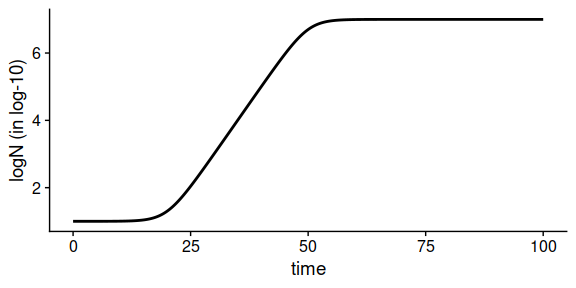Note that the y-axis represents the logarithm of the population size. By default, the label of the y-axis shows within parenthesis the base of the logarithm. This, as well as many other aesthetics of the plot, can be edited by passing additional arguments to plot plot(my_prediction, label_y1 = "log10 of the population size", label_x = "Time (years)", line_size = 2, line_col = "red", line_type = "dotted")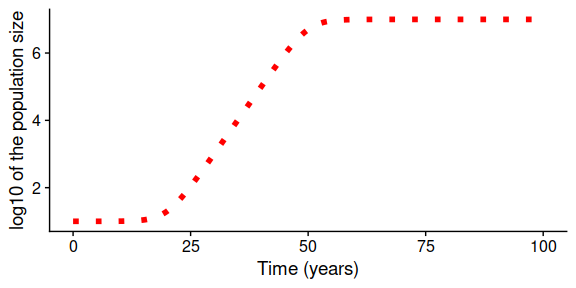For a detailed description of the arguments admitted by the function, please check its help page (e.g. with ?plot.GrowthPrediction). Note that plot() returns an instance of ggplot, so it can be edited using additional layers plot(my_prediction) + theme_gray() + xlab("Time (years)")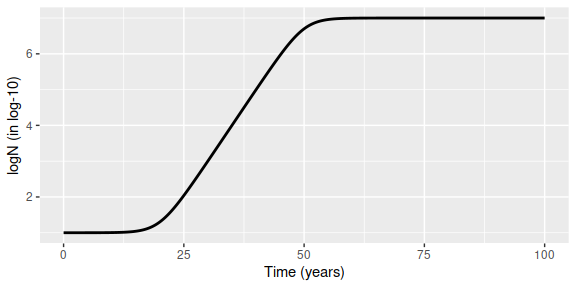One aspect that is often of interest when analyzing the growth of populations is the time required to reach a value of the population size. In biogrowth, this can be calculated using time_to_size(). This function can take as arguments an instance of GrowthPrediction and a population size (in log units) and returns the time required to reach that population size. time_to_size(my_prediction, 3) #>  29.97839 If the population size is not reached during the simulation, the function returns NA. time_to_size(my_prediction, 9) #>  NA ### Alternative definitions of model parameters In order to facilitate the definition of different types of model, predict_growth() accepts alternative definitions of the model parameters. Namely, * the initial population size can also be described either in log-scale (logN0) or without a log transformation (N0), * the maximum population size in the stationary phase can also be described either in log-scale (logNmax) or without a log transformation (Nmax), * the growth rate during the exponential phase can be named mu_opt instead of mu * the duration of the lag phase can be defined in terms of Q0 instead of lambda. In this case, the duration of the lag phase is calculated as $$\lambda=1/\left( \exp(\mu \cdot \lambda) - 1 \right)$$, as per the Baranyi model (see vignette about the mathematical models for the derivation). Note that this calculation is included in biogrowth in the function lambda_to_Q0(). Therefore, this model definition is equivalent to the one defined above: primary_model <- list(model = my_model, logN0 = 1, logNmax = 7, mu = .2, lambda = 20) Q0 <- lambda_to_Q0(lambda = 20, mu = .2) equivalent_pars <- list(model = my_model, N0 = 10^1, Nmax = 10^7, mu_opt = .2, Q0 = Q0) equivalent_prediction <- predict_growth(environment = "constant", my_times, equivalent_pars) plot(equivalent_prediction)### Definition of different logarithmic bases As already mentioned above, growth simulations often cover several orders of magnitude, making it more convenient to express the results in logarithmic scale. By default, all the calculations in biogrowth are done in log10 scale. Nevertheless, this can be modified using the arguments logbase_mu and logbase_logN. We believe the relevance of these bases is a common source of confusion when modeling population growth (although the population size has no units, its logarithmic base affects the calculations), so the reader is strongly encouraged to check the vignette dedicated to the unit system. The base of the logarithm of the population size is defined by logbase_logN. By default, the calculations are done in log10 scale, but any numeric value can be passed to this argument. One point worth highlighting here is that the model parameters logN0 and logNmax are defined in the same scale as the population size. Therefore, if the primary model is defined based on this parameters, the growth curve is exactly the same, regardless of the base of $$\log N$$. The only difference is the label of the y-axis. primary_model <- list(model = my_model, logN0 = 1, logNmax = 7, mu = .2, lambda = 20) prediction_base_e <- predict_growth(environment = "constant", my_times, primary_model, logbase_logN = exp(1)) prediction_base_e #> Growth prediction based on primary models #> #> Growth model: Baranyi #> #> Parameters of the primary model: #> logN0 logNmax mu lambda #> 1.0 7.0 0.2 20.0 #> #> Parameter mu defined in log-e scale #> Population size defined in log-e scale plot(prediction_base_e)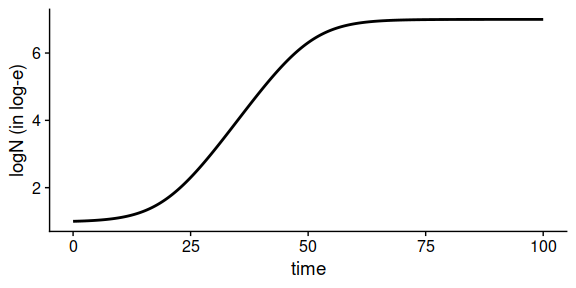However, if the primary model is defined in terms of $$N_0$$ and/or $$N_{max}$$, the growth curve will be affected. primary_model <- list(model = my_model, N0 = 1, Nmax = 1e7, mu = .2, lambda = 20) prediction_base_e <- predict_growth(environment = "constant", my_times, primary_model, logbase_logN = exp(1)) plot(prediction_base_e)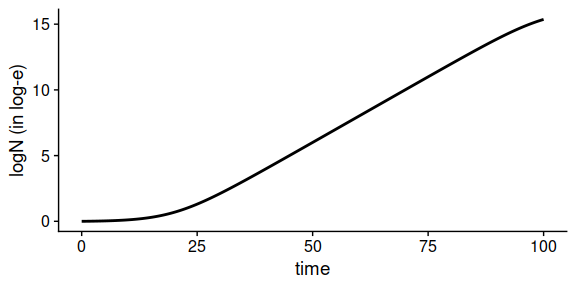The base of the logarithm for the parameter $$\mu$$ is defined by the argument logbase_mu. By default, this parameter uses the same base as the population size. Nonetheless, it can accept any numeric value. Changes in this base will be reflected both in the print method and the growth curve: primary_model <- list(model = my_model, logN0 = 1, logNmax = 7, mu = .2, lambda = 20) prediction_mu_e <- predict_growth(environment = "constant", my_times, primary_model, logbase_mu = exp(1)) prediction_mu_e #> Growth prediction based on primary models #> #> Growth model: Baranyi #> #> Parameters of the primary model: #> logN0 logNmax mu lambda #> 1.0 7.0 0.2 20.0 #> #> Parameter mu defined in log-e scale #> Population size defined in log-10 scale plot(prediction_mu_e)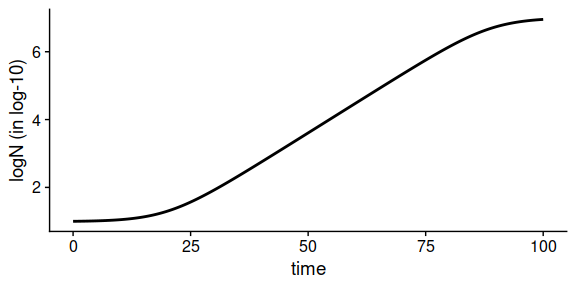As detailed in the vignette dedicated to the unit system, the growth rate for different log-bases of $$\mu$$ can be calculated as $$\mu_b = \mu_a \cdot \log_b a$$ primary_model <- list(model = my_model, logN0 = 1, logNmax = 7, mu = .2 * log(10), lambda = 20) prediction_mu_e <- predict_growth(environment = "constant", my_times, primary_model, logbase_mu = exp(1) ) plot(prediction_mu_e)Note that time_to_size() also accepts a logbase_logN argument. By default, this argument takes NULL, so the function uses the same logbase as the growth prediction. For instance, if we use prediction_base_e that used natural base for the calculation, a size=5 would mean a size of exp(5) units. print(prediction_base_e) #> Growth prediction based on primary models #> #> Growth model: Baranyi #> #> Parameters of the primary model: #> N0 Nmax mu lambda #> 1e+00 1e+07 2e-01 2e+01 #> #> Parameter mu defined in log-e scale #> Population size defined in log-e scale time_to_size(prediction_base_e, size = 5) #>  44.96689 Then, if we want to define the population in log-base10, we would need to set logbase_logN=10. time_to_size(prediction_base_e, log10(exp(5)), logbase_logN = 10) #>  44.96689 ## Growth predictions under dynamic environmental conditions The function predict_growth() can account for the effect of changes in the environmental conditions on parameter $$\mu$$. This behaviour is triggered setting environment="dynamic", and requires the definition of additional arguments: • secondary_models, a nested named list that defines the secondary models (models that describe the effect of the changes of the environmental conditions on $$\mu$$) • env_conditions, a tibble (or data.frame) that describe the variation of the environmental conditions during the experiment. The dynamic environmental conditions are defined using a tibble (or data.frame). It must have a column defining the elapsed time and as many additional columns as needed for each environmental factor. By default, the column defining the time must be called time, although this can be changed using the formula argument. For the simulations, the value of the environmental conditions at time points not included in env_conditions is calculated by linear interpolation. In our simulation we will consider two environmental factors: temperature and pH. We can define their variation using this tibble. To illustrate the use of the formula argument, we will use Time for the column describing the elapsed time. my_conditions <- tibble(Time = c(0, 5, 40), temperature = c(20, 30, 35), pH = c(7, 6.5, 5) ) Then, the simulations would consider this temperature profile ggplot(my_conditions) + geom_line(aes(x = Time, y = temperature))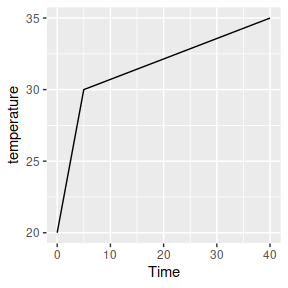And this pH profile ggplot(my_conditions) + geom_line(aes(x = Time, y = pH))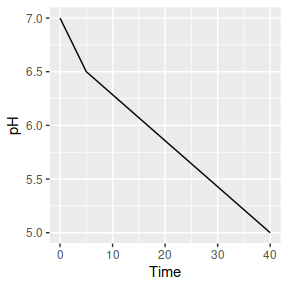We could define smoother profiles using additional rows. For time points outside of the range defined in env_conditions, the value at the closes extreme is used (rule=2 from approx function). For dynamic conditions, biogrowth uses the Baranyi growth model as primary model. This model requires the definition of two model parameters: the specific growth rate at optimum conditions (mu_opt) and the maximum population size (Nmax). Moreover, the initial values of the population size (N0) and the theoretical substance $$Q$$ (Q0) must be defined. Note that $$Q_0$$ is related to the duration of the lag phase under isothermal conditions by the identity $$\lambda = \ln \left( 1 + 1/q_0 \right)/\mu_{max}$$. For the predict_dynamic_growth() function, all variables must be defined in a single list: my_primary <- list(mu_opt = .9, Nmax = 1e8, N0 = 1e0, Q0 = 1e-3) The next step is the definition of the secondary models. In biogrowth, the effect of changes on the environmental conditions in $$\mu$$ are described based on the gamma concept. In this approach, the effect of each environmental condition ($$X_i$$) is considered as a correction factor with respect to the one observed under optimal conditions $$\mu_{opt}$$. Hence, this effect is described by a “gamma” function ($$\gamma_i \left(X_i \right)$$) that takes values between 0 and 1. For additional details on the mathematical methods, the reader is referred to the specific vignette about mathematical methods. $\mu = \mu_{opt} \cdot \gamma_1(X_1) \cdot \gamma_2(X_2) \cdot ... \cdot \gamma_n(X_n)$ Therefore, in this example, we need to define one secondary model per environmental condition. This must be done using a list. This list should contain the type of gamma model as well as the model parameters for each environmental condition. The function secondary_model_data() can aid in the definition of the secondary models. Calling it without any arguments returns the available model keys. secondary_model_data() #>  "CPM" "Zwietering" "fullRatkowsky" For instance, we will define a gamma-type model for temperature as defined by Zwietering et al. (1992). This is done by including an item called model in the list and assigning it the value "Zwietering". Then, we define the values of the model parameters. In a similar way as for primary models, passing a model to secondary_model_data() returns meta-information of the model, including the parameter keys secondary_model_data("Zwietering")$pars
#>  "xmin" "xopt" "n"

In this case, we need to define parameters xmin, xopt and n (for a detailed description of the modeling approach, please check the relevant vignette). We define them using individual entries in the list:

sec_temperature <- list(model = "Zwietering",
xmin = 25,
xopt = 35,
n = 1)

Next, we will define a CPM model for the effect of pH. Note that the model selection is for illustration purposes, not based on any scientific knowledge. First of all, we need to set the item model to "CPM". Then, we need to define the model parameters (note that this model also needs xmax).

sec_pH <- list(model = "CPM",
xmin = 5.5,
xopt = 6.5,
xmax = 7.5,
n = 2)

The final step for the definition of the gamma-type secondary model is gathering all the individual models together in a single list and assigning them to environmental factors. Each element on the list must be named using the same column names as in env_conditions. Before, we had used the column names temperature and pH. Thus

my_secondary <- list(
temperature = sec_temperature,
pH = sec_pH
)

The final argument is the time points where to make the calculations. We can use a numeric vector with 1000 points between 0 and 50 for this:

my_times <- seq(0, 50, length = 1000)

Once we have defined every argument, we can call the predict_dynamic_growth() function. Because we are using Time to define the elapsed time in env_conditions, we must also define the .~Time in the formula argument.

dynamic_prediction <- predict_growth(environment = "dynamic",
my_times,
my_primary,
my_secondary,
my_conditions,
formula = . ~ Time
)

The function returns an instance of GrowthPrediction with the results of the simulation. It includes several S3 methods to ease the interpretation of the results. The print method shows the models used, as well as the environmental factors considered in the simulation. The print method also shows the log-bases used for the calculations. By default, the functions uses log10 base, but this can be modified using logbase_mu and logbase_logN as in simulations for constant environmental conditions.

dynamic_prediction
#> Growth prediction under dynamic environmental conditions
#>
#> Environmental factors included: temperature, pH
#>
#> Parameters of the Baranyi primary model:
#> mu_opt   Nmax     N0     Q0
#>  9e-01  1e+08  1e+00  1e-03
#>
#> Parameter mu defined in log-10 scale
#>
#> Population size defined in log-10 scale
#>
#> Secondary model for temperature:
#>        model         xmin         xopt            n
#> "Zwietering"         "25"         "35"          "1"
#>
#> Secondary model for pH:
#> model  xmin  xopt  xmax     n
#> "CPM" "5.5" "6.5" "7.5"   "2"

Again, the class includes plot methods to visualize the growth curve.

plot(dynamic_prediction)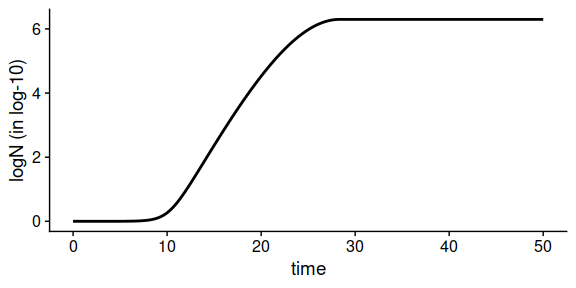This plot can include the variation of a single environmental factor alongside the growth curve. As well as above, several aesthetics of the plot can be modified passing additional arguments to the plotting function. Please check the help page of plot.GrowthPrediction for a complete list of options.

plot(dynamic_prediction,
label_y2 = "Temperature (ºC)")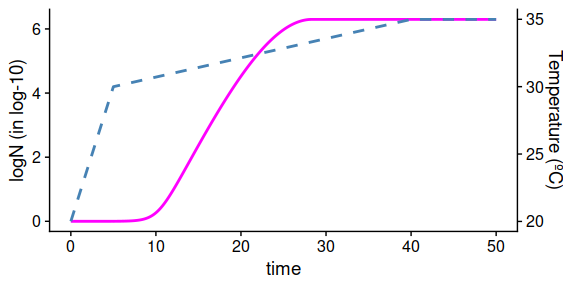Similar to predictions under constant environmental conditions, time_to_size can estimate the time required to reach a given population size:
time_to_size(dynamic_prediction, 3)
#>  16.36868